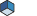Open Inventor Release 2023.2.1
Searching...
No Matches
SbVec2d Class Reference2D vector class (double precision). More...

`#include <Inventor/SbVec.h>`

## Public Member Functions

SbVec2d ()
Default constructor.

SbVec2d (const double v)
Constructor given vector components.

SbVec2d (double x, double y)
Constructor given vector components.

double dot (const SbVec2d &v) const
Returns dot (inner) product of vector and another vector.

const double * getValue () const
Returns vector components.

void getValue (double &x, double &y) const
Returns vector components.

double length () const
Returns geometric length of vector.

double lengthSquared () const
Returns square length of vector.

void negate ()
Negates each component of vector in place.

double normalize ()
Changes vector to be unit length.

SbVec2dsetValue (const double v)
Sets the vector components.

SbVec2dsetValue (double x, double y)
Sets the vector components.

SbVec2dsetValue (const SbVec2f &vec2f)
Sets value of vector from a single precision vector.

double & operator[] (int i)
Accesses indexed component of vector.

const double & operator[] (int i) const

SbVec2doperator*= (double d)
Component-wise scalar multiplication operator.

SbVec2doperator/= (double d)
Component-wise scalar division operator.

SbVec2doperator+= (const SbVec2d &u)

SbVec2doperator-= (const SbVec2d &u)
Component-wise vector subtraction operator.

SbVec2d operator- () const
Nondestructive unary negation - returns a new vector.

SbBool equals (const SbVec2d &v, double tolerance) const
Equality comparison within given tolerance - the square of the length of the maximum distance between the two vectors.

template<typename T >
SbVec2d (const T &v)
Constructor that converts an arbitrary SbVec2 to an SbVec2d.

## Friends

SbVec2d operator* (const SbVec2d &v, double d)
Component-wise binary scalar multiplication operator.

SbVec2d operator* (double d, const SbVec2d &v)
Component-wise binary scalar multiplication operator.

SbVec2d operator/ (const SbVec2d &v, double d)
Component-wise binary scalar division operator.

SbVec2d operator+ (const SbVec2d &v1, const SbVec2d &v2)

SbVec2d operator- (const SbVec2d &v1, const SbVec2d &v2)
Component-wise binary vector subtraction operator.

int operator== (const SbVec2d &v1, const SbVec2d &v2)
Equality comparison operator.

int operator!= (const SbVec2d &v1, const SbVec2d &v2)
Inequality comparison operator.

std::ostream & operator<< (std::ostream &os, const SbVec2d &v)
Writes the vector to the specified output stream.

## Detailed Description2D vector class (double precision).

2D vector class used to store 2D vectors and points using double precision values. Although Open Inventor fields still store only single precision values, for certain applications it is useful and convenient to be able to store and manipulate double precision values, for example, double precision coordinate data or values that will be used for further computation.

Definition at line 314 of file SbVec.h.

## ◆ SbVec2d() [1/4]

 SbVec2d::SbVec2d ( )
inline

Default constructor.

The vector is initialized with zeros.

Definition at line 320 of file SbVec.h.

## ◆ SbVec2d() [2/4]

 SbVec2d::SbVec2d ( const double v )
inlineexplicit

Constructor given vector components.

Definition at line 325 of file SbVec.h.

## ◆ SbVec2d() [3/4]

 SbVec2d::SbVec2d ( double x, double y )
inline

Constructor given vector components.

Definition at line 330 of file SbVec.h.

## ◆ SbVec2d() [4/4]

template<typename T >
 SbVec2d::SbVec2d ( const T & v )
inlineexplicit

Constructor that converts an arbitrary SbVec2 to an SbVec2d.

Definition at line 467 of file SbVec.h.

## ◆ dot()

 double SbVec2d::dot ( const SbVec2d & v ) const
inline

Returns dot (inner) product of vector and another vector.

Definition at line 335 of file SbVec.h.

## ◆ equals()

 SbBool SbVec2d::equals ( const SbVec2d & v, double tolerance ) const

Equality comparison within given tolerance - the square of the length of the maximum distance between the two vectors.

## ◆ getValue() [1/2]

 const double * SbVec2d::getValue ( ) const
inline

Returns vector components.

Definition at line 341 of file SbVec.h.

## ◆ getValue() [2/2]

 void SbVec2d::getValue ( double & x, double & y ) const

Returns vector components.

## ◆ length()

 double SbVec2d::length ( ) const

Returns geometric length of vector.

## ◆ lengthSquared()

 double SbVec2d::lengthSquared ( ) const
inline

Returns square length of vector.

Faster than length().

Definition at line 357 of file SbVec.h.

## ◆ negate()

 void SbVec2d::negate ( )

Negates each component of vector in place.

## ◆ normalize()

 double SbVec2d::normalize ( )

Changes vector to be unit length.

## ◆ operator*=()

 SbVec2d & SbVec2d::operator*= ( double d )

Component-wise scalar multiplication operator.

## ◆ operator+=()

 SbVec2d & SbVec2d::operator+= ( const SbVec2d & u )

## ◆ operator-()

 SbVec2d SbVec2d::operator- ( ) const

Nondestructive unary negation - returns a new vector.

## ◆ operator-=()

 SbVec2d & SbVec2d::operator-= ( const SbVec2d & u )

Component-wise vector subtraction operator.

## ◆ operator/=()

 SbVec2d & SbVec2d::operator/= ( double d )

Component-wise scalar division operator.

## ◆ operator[]() [1/2]

 double & SbVec2d::operator[] ( int i )
inline

Accesses indexed component of vector.

Definition at line 390 of file SbVec.h.

## ◆ operator[]() [2/2]

 const double & SbVec2d::operator[] ( int i ) const
inline

Definition at line 391 of file SbVec.h.

## ◆ setValue() [1/3]

 SbVec2d & SbVec2d::setValue ( const double v )

Sets the vector components.

## ◆ setValue() [2/3]

 SbVec2d & SbVec2d::setValue ( const SbVec2f & vec2f )
inline

Sets value of vector from a single precision vector.

Definition at line 383 of file SbVec.h.

## ◆ setValue() [3/3]

 SbVec2d & SbVec2d::setValue ( double x, double y )

Sets the vector components.

## ◆ operator!=

 int operator!= ( const SbVec2d & v1, const SbVec2d & v2 )
friend

Inequality comparison operator.

Definition at line 449 of file SbVec.h.

## ◆ operator* [1/2]

 SbVec2d operator* ( const SbVec2d & v, double d )
friend

Component-wise binary scalar multiplication operator.

## ◆ operator* [2/2]

 SbVec2d operator* ( double d, const SbVec2d & v )
friend

Component-wise binary scalar multiplication operator.

Definition at line 425 of file SbVec.h.

## ◆ operator+

 SbVec2d operator+ ( const SbVec2d & v1, const SbVec2d & v2 )
friend

## ◆ operator-

 SbVec2d operator- ( const SbVec2d & v1, const SbVec2d & v2 )
friend

Component-wise binary vector subtraction operator.

## ◆ operator/

 SbVec2d operator/ ( const SbVec2d & v, double d )
friend

Component-wise binary scalar division operator.

## ◆ operator<<

 std::ostream & operator<< ( std::ostream & os, const SbVec2d & v )
friend

Writes the vector to the specified output stream.

Definition at line 3620 of file SbVec.h.

## ◆ operator==

 int operator== ( const SbVec2d & v1, const SbVec2d & v2 )
friend

Equality comparison operator.

The documentation for this class was generated from the following file: